# eigenvalues

## The sum of the two largest eigenvalues ★★

Author(s): Gernert

Problem   Letbe a graph onvertices and let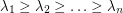be the eigenvalues of. Is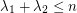?

Keywords: eigenvalues; spectrum

## Alon-Saks-Seymour Conjecture ★★★

Author(s): Alon; Saks; Seymour

Conjecture   Ifis a simple graph which can be written as an union ofedge-disjoint complete bipartite graphs, then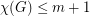.

## Fowler's Conjecture on eigenvalues of (3,6)-polyhedra ★★

Author(s): Fowler

Conjecture   Letbe the graph of a-polyhedron with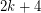vertices. Then the eigenvalues ofcan be partitioned into three classes:,(whereis nonnegative for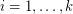), and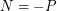.

Keywords: (3,6)-polyhedron; eigenvalues